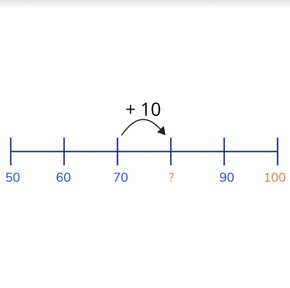Skip counting by tens to 100 from multiples of ten

# Skip counting by tens to 100 from multiples of ten

Learn to skip count by tens to 100 from multiples of ten.

No account needed.8,000 schools use Gynzy92,000 teachers use Gynzy1,600,000 students use Gynzy

## General

Students learn to skip count by tens to 100 from multiples of ten.

## Standards

CCSS.Math.Content.K.CC.A.1

## Learning objective

Students will be able to skip count by tens to any number up to 100 from multiples of ten.

## Introduction

There is a puzzle visible on the interactive whiteboard. Ask students to drag the puzzle pieces from the written number to the number as digits. If they do this correctly, they should complete the puzzle.

## Instruction

Explain that you are going to skip count by tens. A tens number ends with a 0 in the ones place. By making jumps of 10, you jump from one tens number to the next tens number, which is 10 more. Count from 10 to 100 with jumps of 10. Point at the numbers and ask students to count along. Show the number line which shows the jumps of ten. Ask students if they can determine which numbers belong at the question marks. Then students are asked to skip count forward and to drag the numbers to where they belong. Then tell students that you can also skip count backward by tens. Point at the numbers and skip count backward by tens from 100 to 0. Show them the number line and ask students which numbers belong at the question marks. As a class, practice skip counting backward and fill in the missing numbers. Then practice skip counting forward and backward as a class, and have students write the missing numbers on a sheet of paper or mini whiteboard, and hold up their answers to check their work.

Check that students are able to skip count by tens to 100 from multiples of ten by asking the following questions:
- How do you skip count by tens?
- Skip count forward by 10s from 20 to 100.
- Skip count backward by 10s from 70 and count to 0.

## Quiz

Students are given a selection of questions in which they must skip count forward by tens, and backward by tens. They are first supported by a number line, and later are asked to count independently.

## Closing

Remind students that it is important to be able to skip count by tens to 100 from a multiple of ten so you can quickly count to any number. Ask five students to come to the front of the class and give them five consecutive number cards which have tens numbers (up to 100). Ask the students to get into numerical order. Once they have correctly lined up, ask the class to determine which numbers come before the smallest number and which number comes after the largest number. They do so by skip counting forward and backward by 10. You can repeat this with 5 more students.

## Teaching tips

Students who have difficulty with this learning goal can be supported by making use of a number line on which they can track their progress. They can always double check their skip counting by counting one by one. Have them practice skip counting forward before working on skip counting backward.

## Instruction materials

Number cards in multiples of ten from 0-100.

### The online teaching platform for interactive whiteboards and displays in schools

• Save time building lessons

• Manage the classroom more efficiently

• Increase student engagement